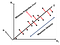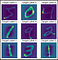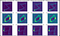# Principle Components Analysis (PCA): Essence and Case Study with Python

Data is the fuel of big data era, and we can get insightful information from data. However, tons of data in a high number of dimensions may cover valuable knowledge. Therefore, data mining and feature engineering become essential skills to uncover valuable information underneath the data.

## 1. What is PCA

For the dimensionality reduction, Principle Component Analysis (PCA) is the most popular algorithm. PCA is an algorithm encoding the original features into a compact representation and we can drop the “unimportant” features while still retaining most of useful information. PCA does not simply select the useful features and discard the others from original data set, the principal components resulting from PCA are linear combinations of original features and these components are good alternative to represent the original data.

## 2. Essence of PCA

The principle of PCA algorithm is to create a set of new features based on raw data, and rank the order of variance of new feature, finally create a set of principle components. Why the variance is considered as the most important index, it is because more variance in feature values can provide better predicting ability for machine learning model. For example, predicting car price with two features: color and mileage. If all the cars have same color, but with different mileage, then we can not predict car’s price with color (feature without variance). We can only rely on mileage to predict car’s price, the more mileage, the lower car’s price.Fig 1. The basic principle of PCA

## 3. Implement of PCA

From above description, we have a high level understanding about PCA algorithm. It is time to dive into the detailed implementation of PCA.

## 4. Case Study

MNIST data set is used for this project to demonstrate the implement of PCA, meanwhile to illustrate the effects of PCA on high dimension data sets. The data set contains 70000 images of handwritten digits. For each image, the data contains 28x28 = 784 features which represent grayscale level.

`import pandas as pdfrom sklearn.datasets import fetch_openmlfrom sklearn.preprocessing import StandardScalerfrom sklearn.model_selection import train_test_splitfrom sklearn.decomposition import PCA`
`mnist = fetch_openml('mnist_784')for key in mnist:    print (key)datatargetframefeature_namestarget_namesDESCRdetailscategoriesurl`
`X, y = mnist['data'], mnist['target']print(X.shape, y.shape)(70000, 784) (70000,)`
`import matplotlib.pyplot as pltfig = plt.figure( figsize=(6,6) )for i in range(9):    image = X[i]    image_pixels = image.reshape(28,28)    plt.subplot(3,3,i+1)    plt.imshow(image_pixels)    plt.title(f'Target Label {y[i]}', fontsize=12)    plt.axis('off')`The image of first nine data point.
`scaler_train = StandardScaler()scaler_train.fit(X)X = scaler_train.transform(X)`
`pca = PCA(0.95)pca.fit(X)X_pca_reduceddimension = pca.transform(X)pca.n_components_332X_pca_reduceddimension.shape(70000, 332)`
`import matplotlib.pyplot as pltfig = plt.figure(figsize=(15,9))m = 0for i in range(3):    for j in [1, 0.95, 0.85, 0.75]:        m += 1        pca = PCA(j)        pca.fit(X)        X_pca = pca.transform(X)        components = pca.n_components_        X_approx = pca.inverse_transform(X_pca)        plt.subplot(3,4,m)        if j == 1:            image = X[i]            plt.title(f'Original Data; Components 784')        else:             image = X_approx[i]            plt.title(f'Variance {j}; Components {components}')        image_pixels = image.reshape(28,28)        plt.axis('off')        plt.imshow(image_pixels)`Compare different image keeping different original information (variance)

## 5. Summary

This post mainly explained the principal of PCA, meanwhile demonstrated the implements and application of PCA. PCA is one powerful tool to reduce high dimension data set while still keep the majority of data information. If we can properly utilize PCA, it will save us computing resources and time.

Data Scientist Costar

## More from Xia Song

Data Scientist Costar